Swift Package Manager… SPM… It is everywhere, many use it and it is most likely the future of working with Swift dependencies. A single file to fetch all the sweet Open Source packages. And with higher acceptance of the community, even more packages will get available without installing any more tools, such as Cocoapods or Carthage.

But how can we leverage this dependency structure even further? Is external code the only reason for using a package manager?

Our codebases are growing with every single new file. First, we create a folder structure to organize our .swift files, but then even the slightest code requires Xcode to recompile everything. Our build process becomes slower… and slower… and ….… goes away to grab a coffee while waiting for Xcode to finish compilation ….… slower.

Even worse when working with feature-rich, large-scale apps. They become clunky and you spend a lot of time waiting for rebuilding unchanged parts when you just want to iterate your own new, fresh feature.

Example:

A feature-rich receipt tracking app, which connects to your bank account for matching transactions, uses a cloud for live synchronization, sharing accounts with friends, etc. You want to add a scanning feature, which takes a photo and converts it into the receipt data your app uses.

## SPM to the rescue!

Swift Package Manager allows us to create small, reusable code packages. On the one hand, this allows us to isolate unchanged code during the build process, and on the other hand, it allows us to simply create a spin-off demo version of the app, with only the necessary parts to improve a single feature.

Continuing the example above: Using local SPM packages, you can create a small prototyping app that only shows the scan feature. When the feature is done, it can be used in the main app.

Let me give you a quick overview of how we are going to build our own multi-platform *Calculator *as an iOS app and a command-line tool (the guide for creating the iOS app can be applied for macOS too):

1. Create a starter SPM command-line tool
2. Moving logic code into own SPM library
3. Create the iOS project using the library
4. Create more local libraries to build a dependency graph

If you are more interested in the final solution, check out this GitHub repository for the final code.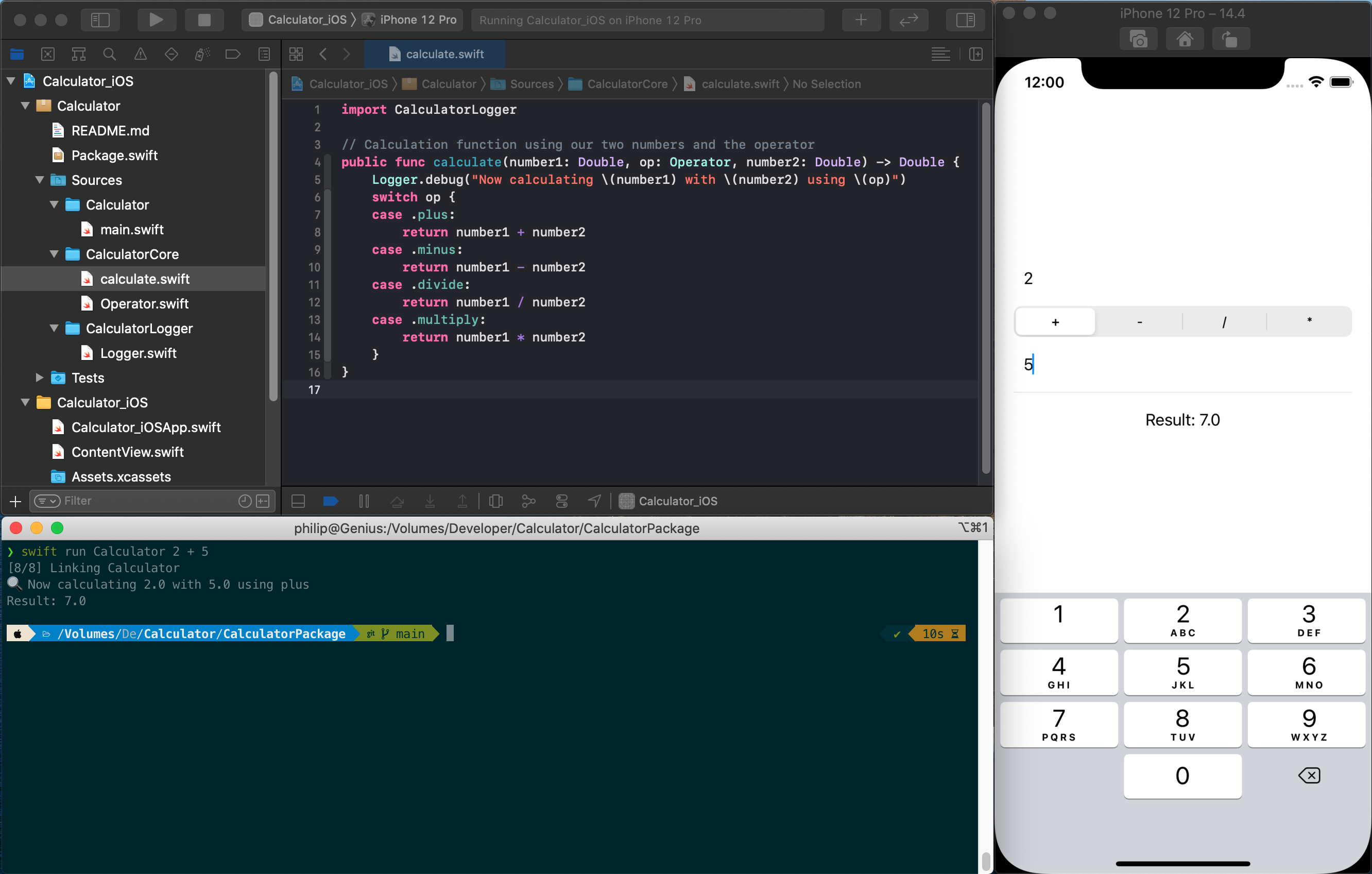iOS app and command-line executable offering the same functionality

## Creating an SPM command-line tool

Start off launching your Terminal of choice (for me it’s iTerm2). Then go ahead and create a new folder called Calculator and afterward change the working directory into that folder:

``````\$ mkdir Calculator
\$ cd Calculator
``````

The next step is initializing our Swift package. The Swift Command Line Interface (CLI) allows us to create multiple types of packages. To figure which ones, run swift package init –help for a list:

``````\$ swift package init --help
OVERVIEW: Initialize a new package

OPTIONS:
--name   Provide custom package name
--type   empty|library|executable|system-module|manifest
``````

Our main focus is on the library and executable. If you are just creating a library package, run `swift package init --type library` . But in our case, we want to start with an executable (leading \$ means it is a command):

``````\$ swift package init --type executable
Creating executable package: Calculator
Creating Package.swift
Creating .gitignore
Creating Sources/
Creating Sources/Calculator/main.swift
Creating Tests/
Creating Tests/LinuxMain.swift
Creating Tests/CalculatorTests/
Creating Tests/CalculatorTests/CalculatorTests.swift
Creating Tests/CalculatorTests/XCTestManifests.swift
``````

Awesome! You created your first Swift package 🔥

Our folder structure now looks like the following:

``````Calculator
├── Package.swift
├── Sources
│   └── Calculator
│       └── main.swift
└── Tests
├── CalculatorTests
│   ├── CalculatorTests.swift
│   └── XCTestManifests.swift
└── LinuxMain.swift
``````

To start working, simply open/double-click the `Package.swift` file and Xcode will recognize it as a package(-project).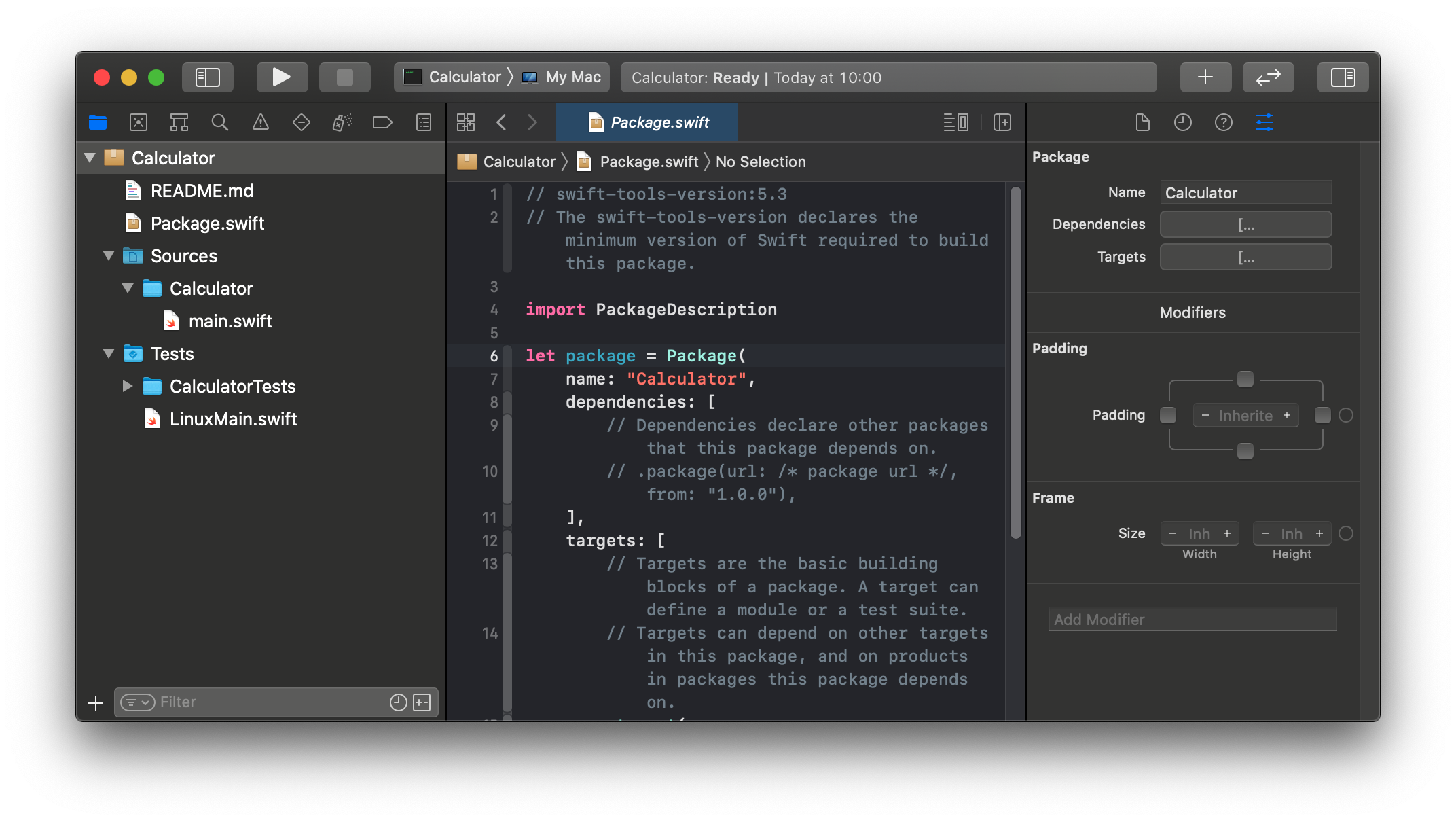As this blog post is not so much of a tutorial about building a calculator in Swift, I am providing you only simple implementation steps in the comments (let me know on Twitter if you want a more detailed tutorial).

Place the following code in your `main.swift` file:

``````import Foundation

let arguments = CommandLine.arguments

// We expect three parameters: first number, operator, second number
func printUsage(message: String) {
let name = URL(string: CommandLine.arguments)!.lastPathComponent
print("usage: " + name + " number1 [+ | - | / | *] number2")
print("    " + message)
}

// The first one is the binary name, so in total 4 arguments
guard arguments.count == 4 else {
printUsage(message: "You need to provide two numbers and an operator")
exit(1);
}
// We expect the first parameter to be a number
guard let number1 = Double(arguments) else {
printUsage(message: arguments + " is not a valid number")
exit(1);
}
// We expect the second parameter, to be one of our operators
enum Operator: String {
case plus = "+"
case minus = "-"
case divide = "/"
case multiply = "*"
}
guard let op = Operator(rawValue: arguments) else {
printUsage(message: arguments + " is not a known operator")
exit(1);
}
// We expect the third parameter to also be a number
guard let number2 = Double(arguments) else {
printUsage(message: arguments + " is not a valid number")
exit(1);
}
// Calculation function using our two numbers and the operator
func calculate(number1: Double, op: Operator, number2: Double) -> Double {
switch op {
case .plus:
return number1 + number2
case .minus:
return number1 - number2
case .divide:
return number1 / number2
case .multiply:
return number1 * number2
}
}
// Calculate the result
let result = calculate(number1: number1, op: op, number2: number2)
// Print result to output
print("Result: \(result)")
``````

To use your new calculator, go back to the terminal and inside the package folder, use the swift run command to test the implementation:

``````\$ swift run Calculator 13 + 14
Result: 27.0
``````

## Moving logic code into own SPM library

We got the first of our two applications up and running. Before we continue to create the iOS app, let’s review the code and figure out, which parts should be shared by all the applications.

Two parts of the code are relevant:

• The enum Operator which is our collection of math operators
• The calculate function is taking two numbers and an operator to perform the actual math.

So let’s start by creating a new library. First off we clean up the default `Package.swift` manifest file by removing all the comments and unused arguments:

``````// swift-tools-version:5.3

import PackageDescription

let package = Package(
name: "Calculator",
targets: [
.target(name: "Calculator"),
.testTarget(name: "CalculatorTests",
dependencies: ["Calculator"]),
]
)
``````

Now create a new folder inside the Sources folder called CalculatorCore which is our shared application core logic.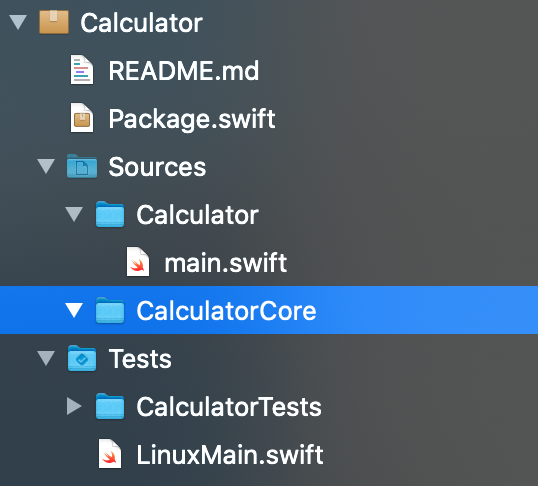Inside this newly created folder we are creating two new Swift files:

First, create the file `Operator.swift` and move the `enum Operator {...}` declaration in there:

``````enum Operator: String {
case plus = "+"
case minus = "-"
case divide = "/"
case multiply = "*"
}
``````

Second, create another file `calculate.swift` and move the calculate(…) function into there:

``````// Calculation function using our two numbers and the operator
func calculate(number1: Double, op: Operator, number2: Double) -> Double {
switch op {
case .plus:
return number1 + number2
case .minus:
return number1 - number2
case .divide:
return number1 / number2
case .multiply:
return number1 * number2
}
}
``````

After moving out the code, your `main.swift` file should now look like this:

``````import Darwin
import Foundation

let arguments = CommandLine.arguments

// We expect three parameters: first number, operator, second number
func printUsage(message: String) {
let name = URL(string: CommandLine.arguments)!.lastPathComponent
print("usage: " + name + " number1 [+ | - | / | *] number2")
print("    " + message)
}

// The first one is the binary name, so in total 4 arguments
guard arguments.count == 4 else {
printUsage(message: "You need to provide two numbers and an operator")
exit(1);
}
// We expect the first parameter to be a number
guard let number1 = Double(arguments) else {
printUsage(message: arguments + " is not a valid number")
exit(1);
}
// We expect the second parameter, to be one of our operators
guard let op = Operator(rawValue: arguments) else {
printUsage(message: arguments + " is not a known operator")
exit(1);
}
// We expect the third parameter to also be a number
guard let number2 = Double(arguments) else {
printUsage(message: arguments + " is not a valid number")
exit(1);
}
// Calculate the result
let result = calculate(number1: number1, op: op, number2: number2)
// Print result to output
print("Result: \(result)")
``````

If you try to run your application once again, it will greet you with an error saying it can’t find type `Operator` nor the function `calculate` anymore.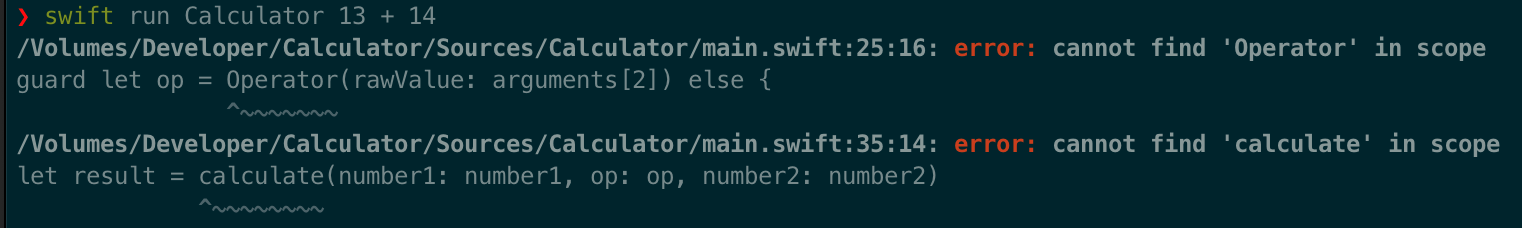This is expected, so now we have to finish creating the library `CalculatorCore` and add it as a dependency to our app target `Calculator`. To do so, all we need is to declare the library in our `Package.swift` :

``````// swift-tools-version:5.3

import PackageDescription

let package = Package(
name: "Calculator",
targets: [
.target(name: "CalculatorCore"),
.target(name: "Calculator",
dependencies: ["CalculatorCore"]),
.testTarget(name: "CalculatorTests",
dependencies: ["Calculator"]),
]
)
``````

If you try to run the application once more, you will still see the same errors. The reason behind this behavior is the missing `import CalculatorCore` in the `main.swift`:

``````import Foundation
import CalculatorCore

...
``````

Additionally, the (in my opinion great) isolation capabilities of Swift packages require us to declare both `Operator` and `calculate` as `public` or otherwise, they won’t be available outside the package:

``````// in Operator.swift:
public enum Operator { ... }
// in calculate.swift:
public func calculate(...) -> Double { ... }
``````

Run your application using swift run and it should be working once again.

## Create the iOS project using the library

Good job so far! You already created a command-line executable and an SPM library. Now we expand it even further and create an iOS app using our SPM library calculation logic.

For this tutorial, we will be using a SwiftUI app, as it is the future of iOS/macOS app development, and allows us to create a simple calculator way faster than using traditional UIKit.

Open up Xcode and click on File/New/Project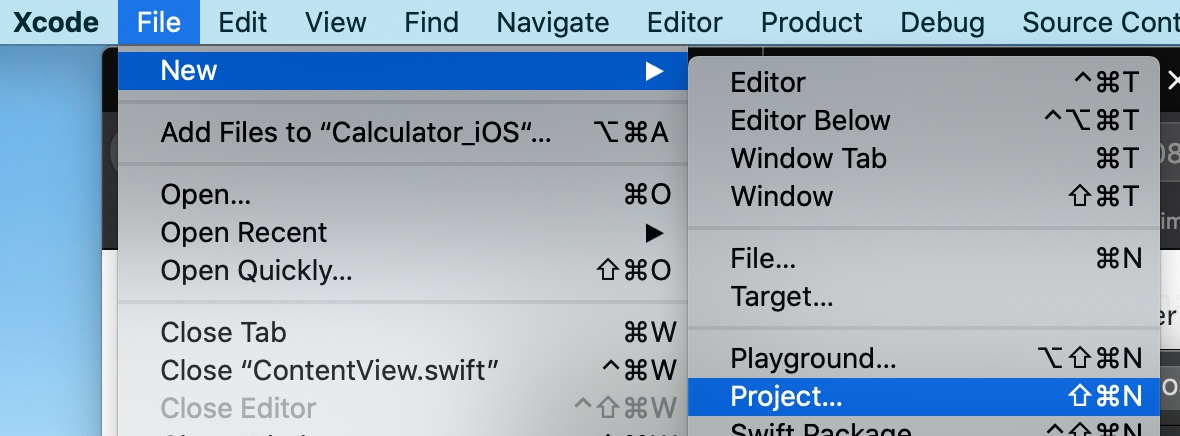Now select App in the iOS tab, name it *Calculator_iOS *and select SwiftUI for all the settings.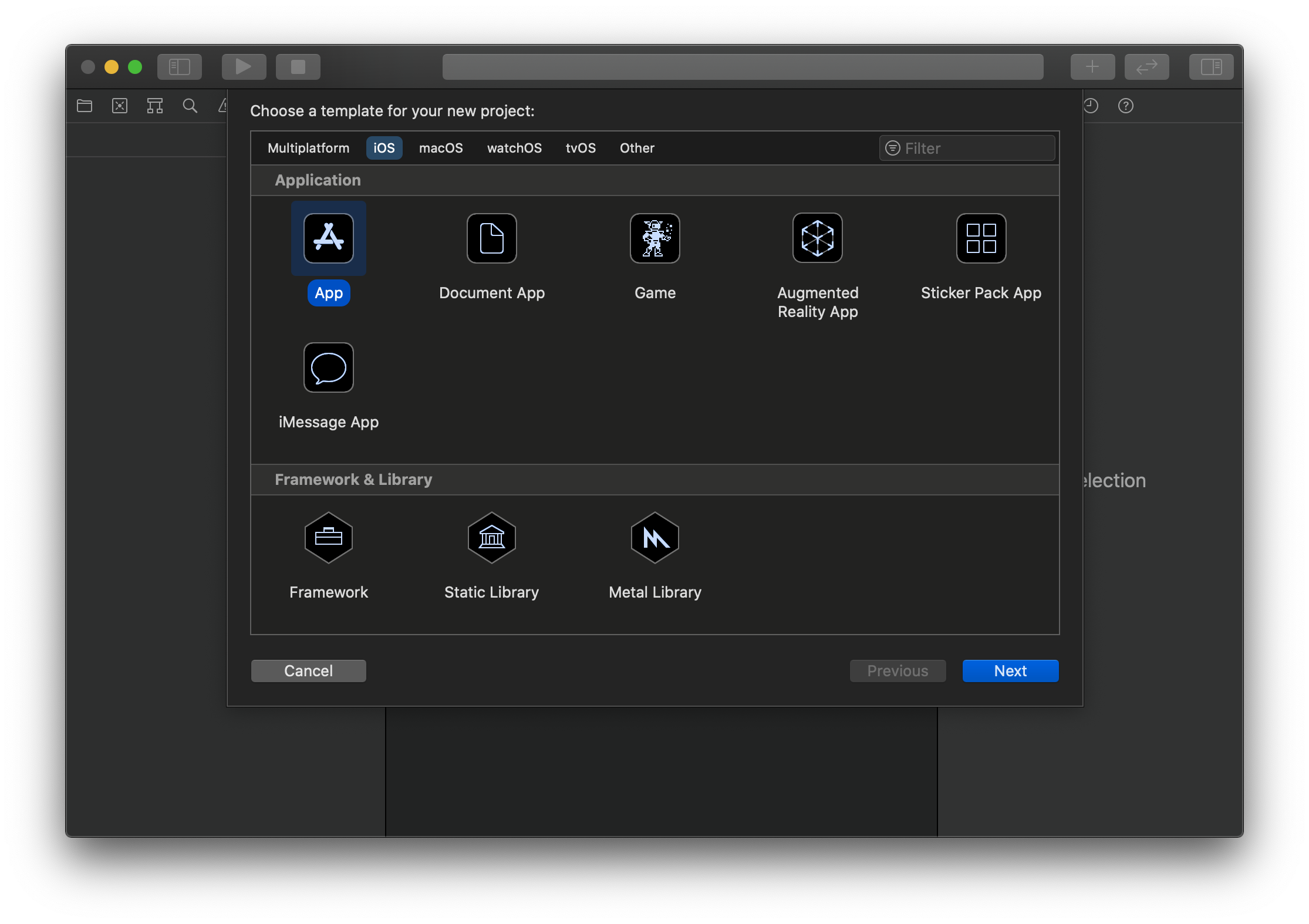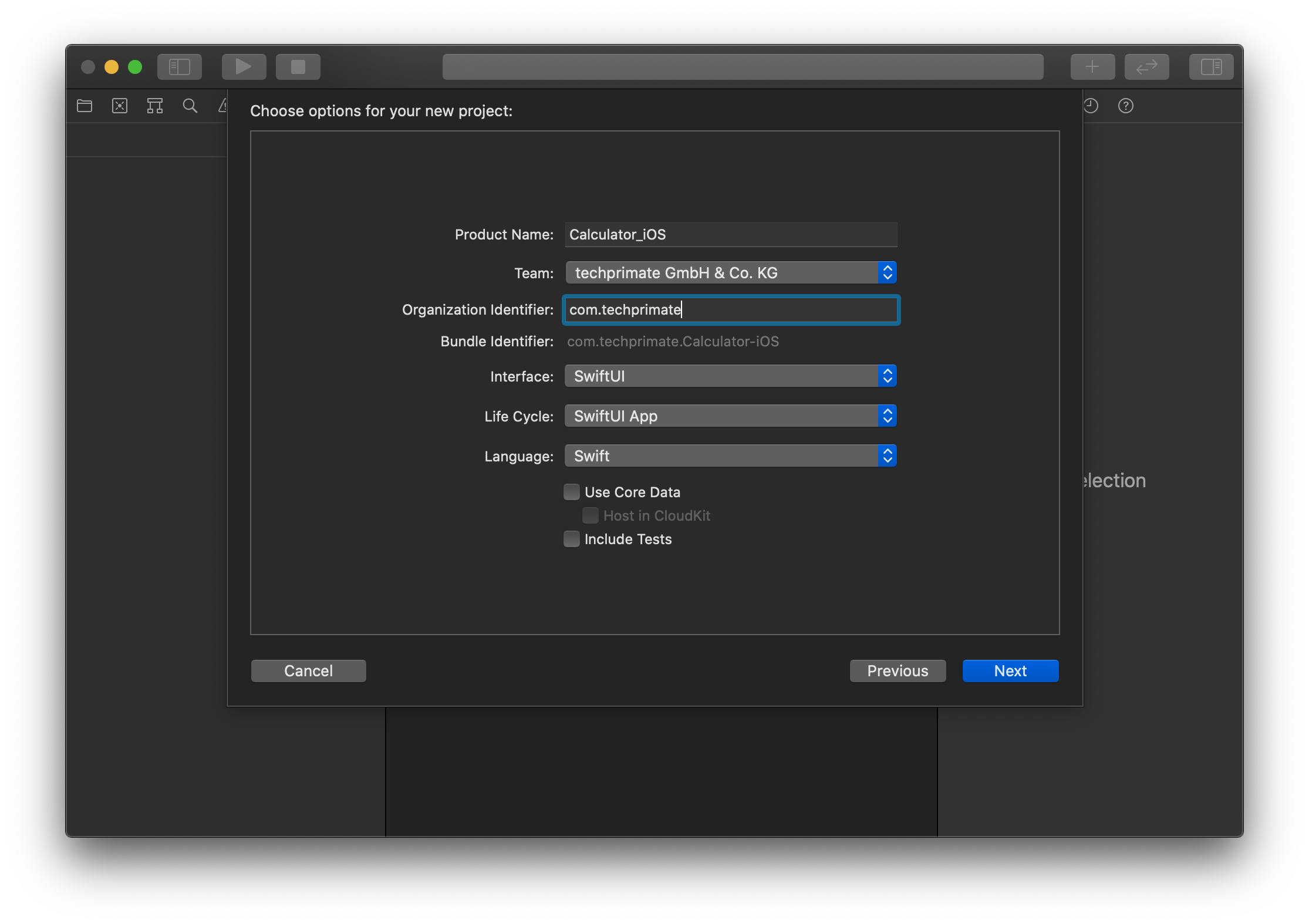Make sure to place the project in your main Calculator folder, and you should end up with the following file structure: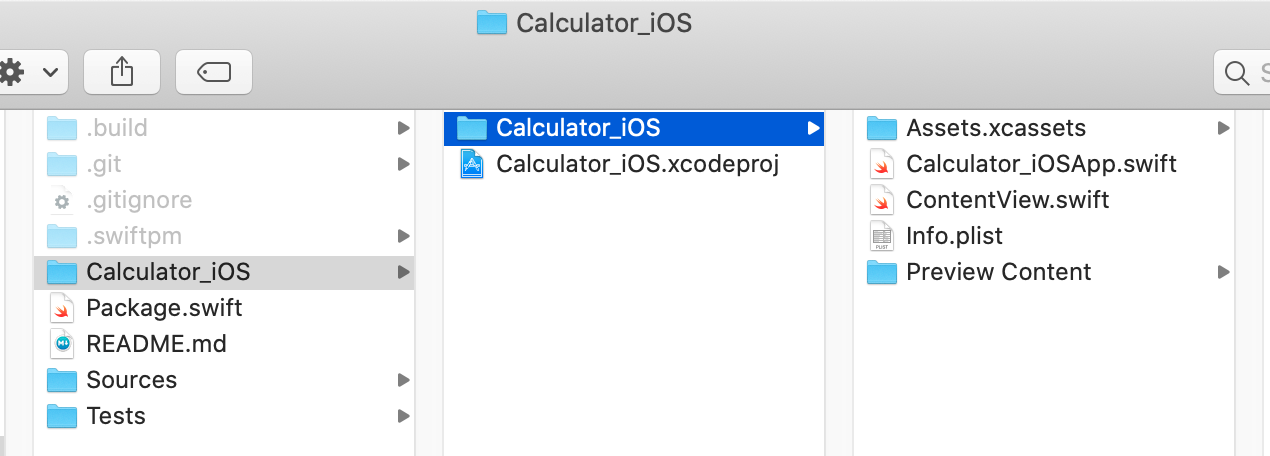As we don’t need the nested iOS folder, close the Xcode project and move the content up one level. Additionally, we move the package into its own subfolder `Calculator`, so afterwards your folder structure should look like this: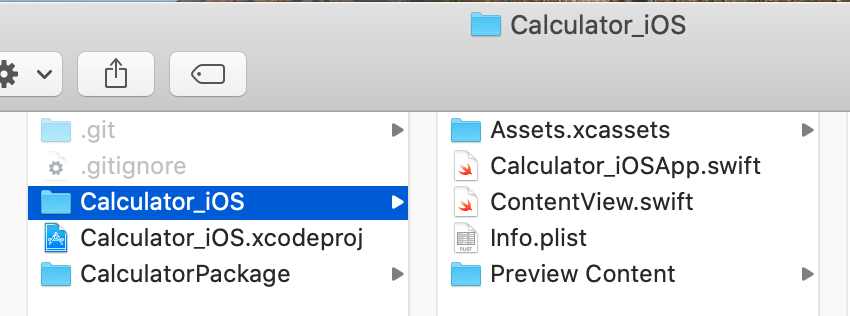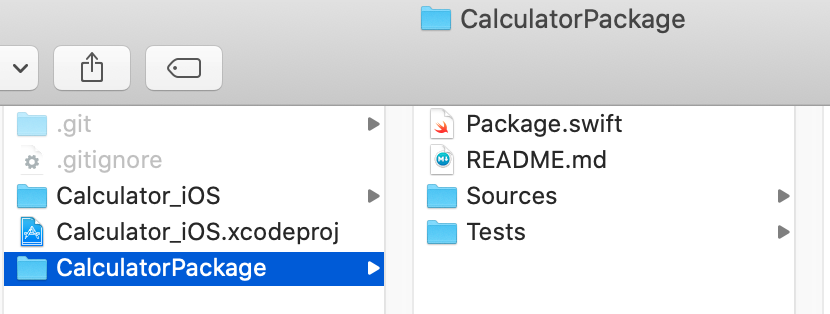Now open up the `Calculator_iOS.xcodeproj`, select your simulator of choice and run the initial application to make sure everything is fine and working.

As the next step, we go ahead and create our calculator UI using two text fields and an operator selection. Replace your struct ContentView {…} inside the ContentView.swift with the following code and run the application once again:

``````struct ContentView: View {

@State var number1 = ""
@State var op = "+"
@State var number2 = ""

var body: some View {
VStack {
TextField("Number 1", text: \$number1)
Picker("Operator", selection: \$op) {
ForEach(["+", "-", "*", "/"], id: \.self) { op in
Text(op)
}
}
.pickerStyle(SegmentedPickerStyle())
TextField("Number 2", text: \$number2)
Divider()
Text("Result: " + result)
}
}

var result: String {
return "?"
}
}
``````

Your basic calculator is done as you can enter numbers and select an operator: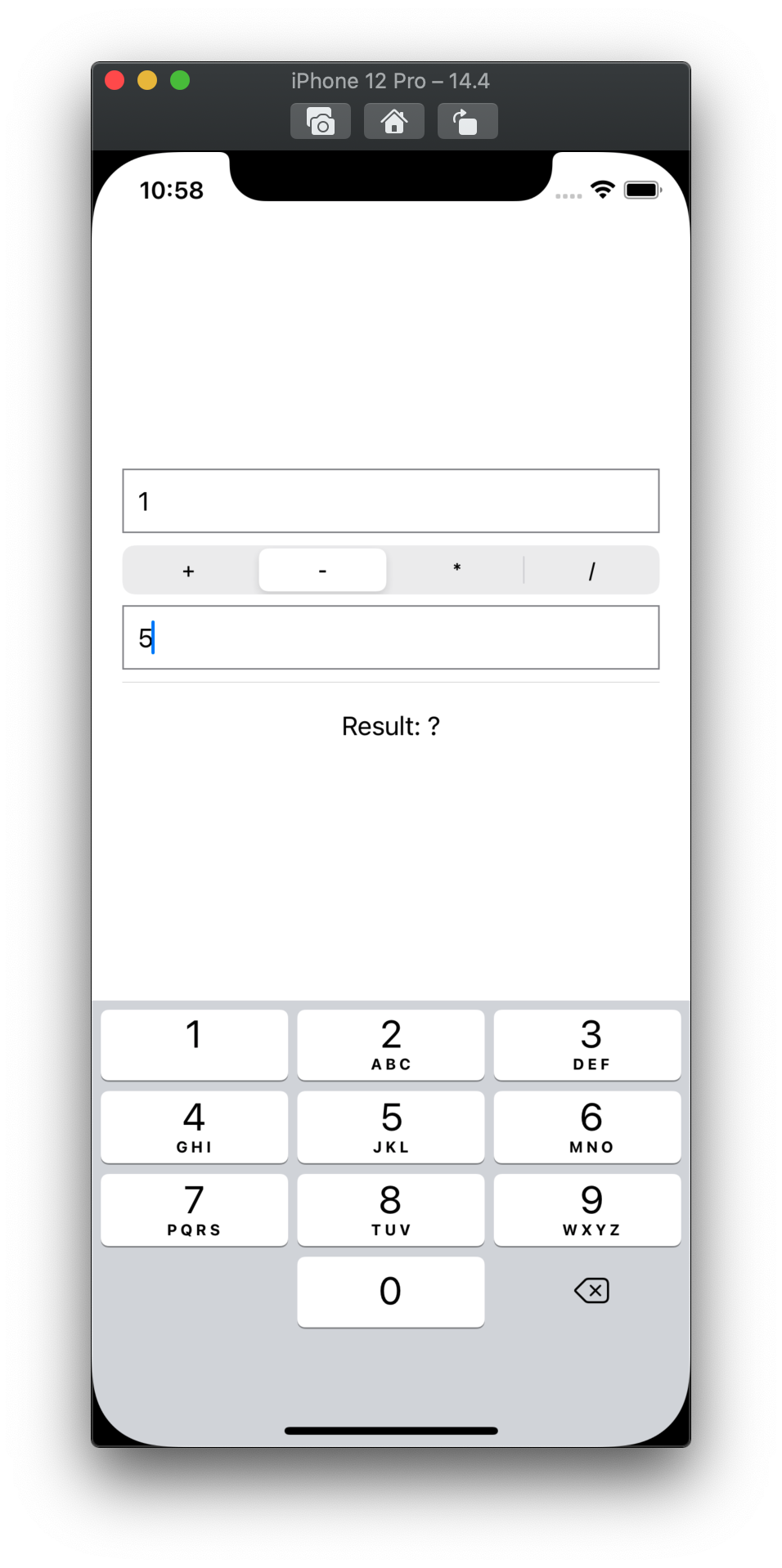Next is adding our local Swift package as an iOS application dependency. This step is not documented or known that well, but very easy. All you have to do is drag the folder CalculatorPackage into the Calculator_iOS file browser at the very top: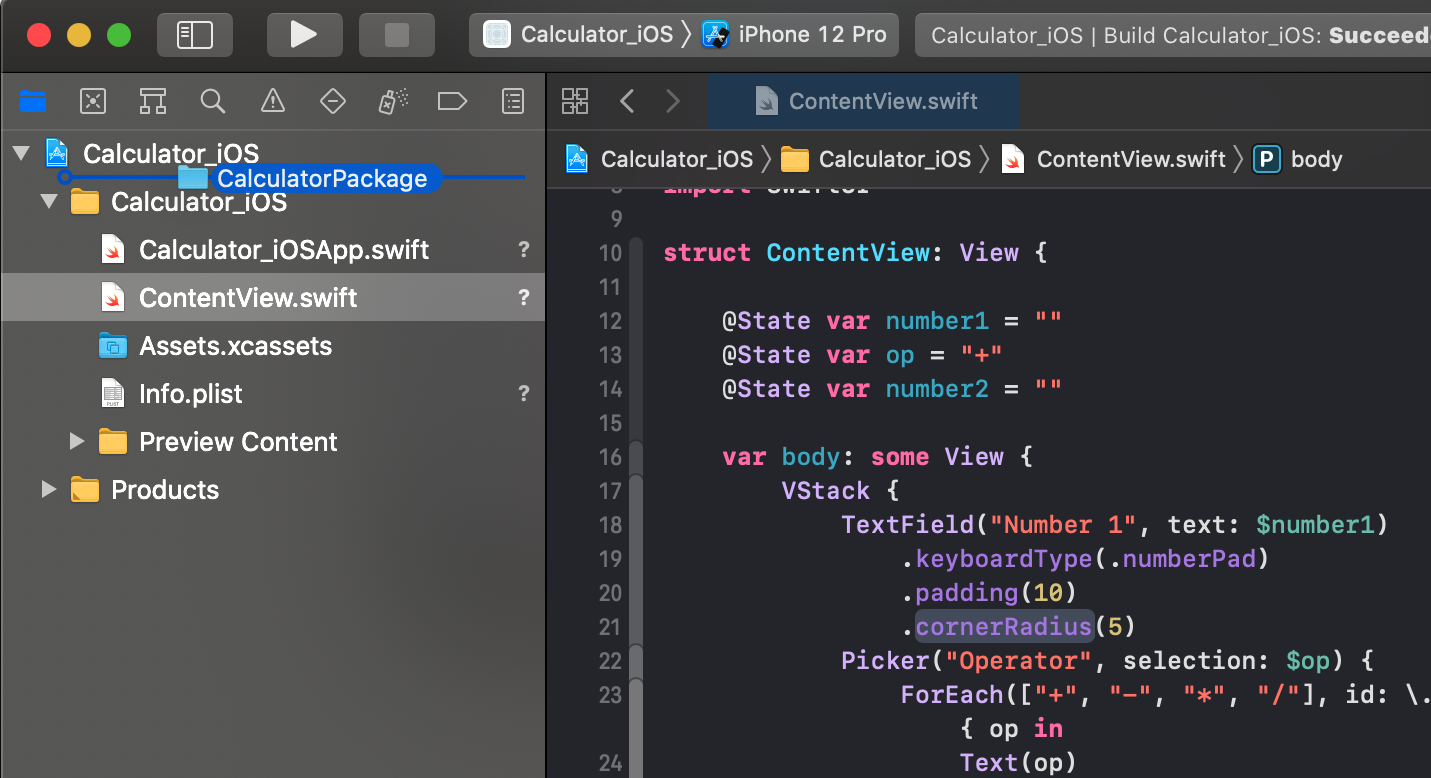And afterwards, Xcode will detect the folder as a local package: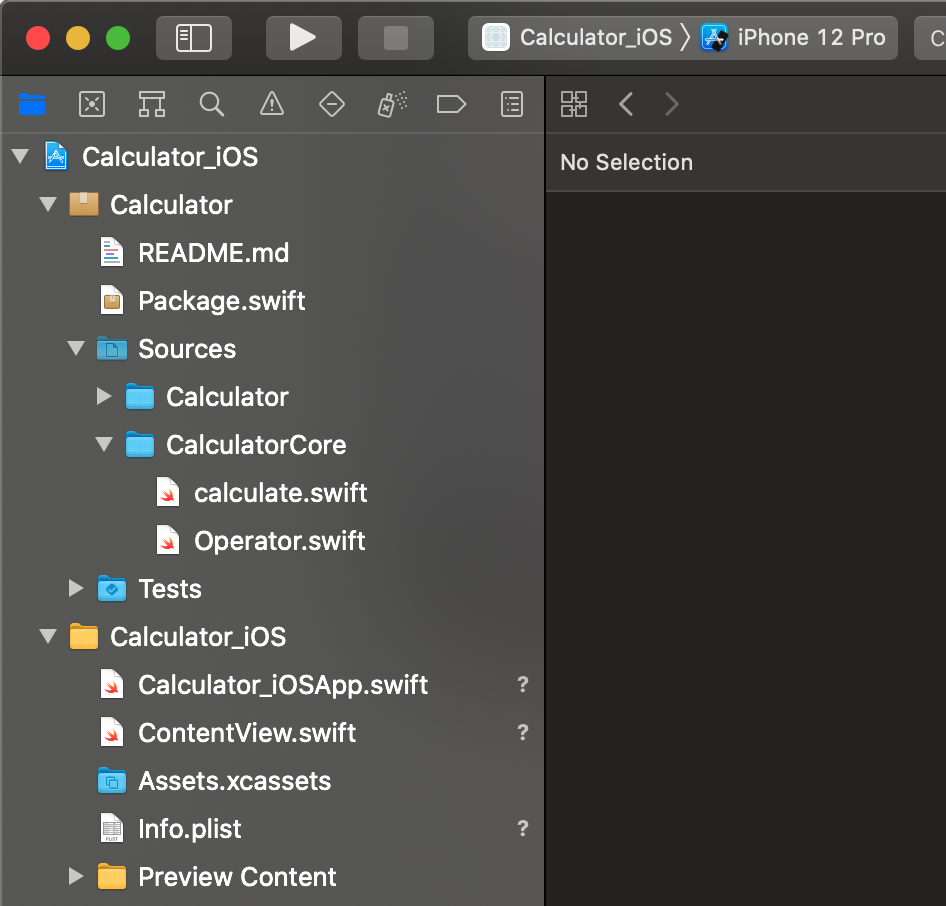Before we can actually add our library to the iOS project, we need to declare it as a product inside the `Package.swift`. As a library product can bundle multiple targets together, we need to add the CalculatorCore as the targets parameter.

``````let package = Package(
name: "Calculator",
products: [
.library(name: "CalculatorCore",
targets: ["CalculatorCore"])
],
targets: [
.target(name: "CalculatorCore"),
.target(name: "Calculator",
dependencies: ["CalculatorCore"]),
.testTarget(name: "CalculatorTests",
dependencies: ["Calculator"]),
]
)
``````

As a final step you have to add the CalculatorCore library as a dependency to the iOS app target, by clicking on the plus + in the target settings in the Frameworks, Libraries, and Embedded Content section and selecting it in the list: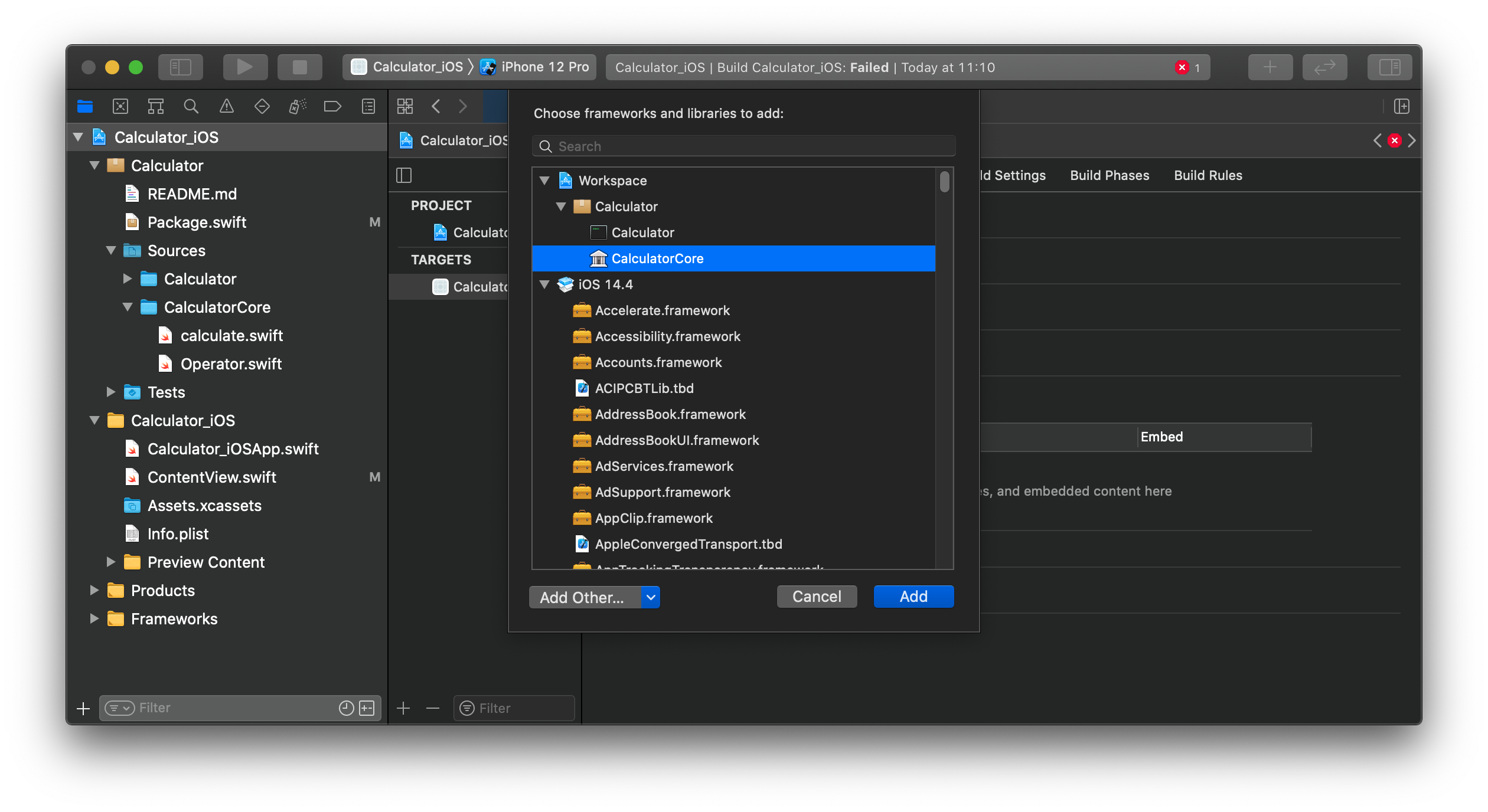This is it. Your local Swift Package is now available inside your iOS app 🎉

Inside the ContentView.swift we can add the import CalculatorCore at the top of the file and once again we can use the `Operator` type and `calculate` function inside the computed property result :

``````var result: String {
guard let num1 = Double(number1) else {
return number1 + " is not a valid number"
}
guard let num2 = Double(number2) else {
return number2 + " is not a valid number"
}
// Force unwrap the operator for now,
// as we can be sure that we only added known ones
let op = Operator(rawValue: self.op)!
let result = calculate(number1: num1, op: op, number2: num2)
return result.description
}
``````

Run the app once again and you can use the calculator inside iOS: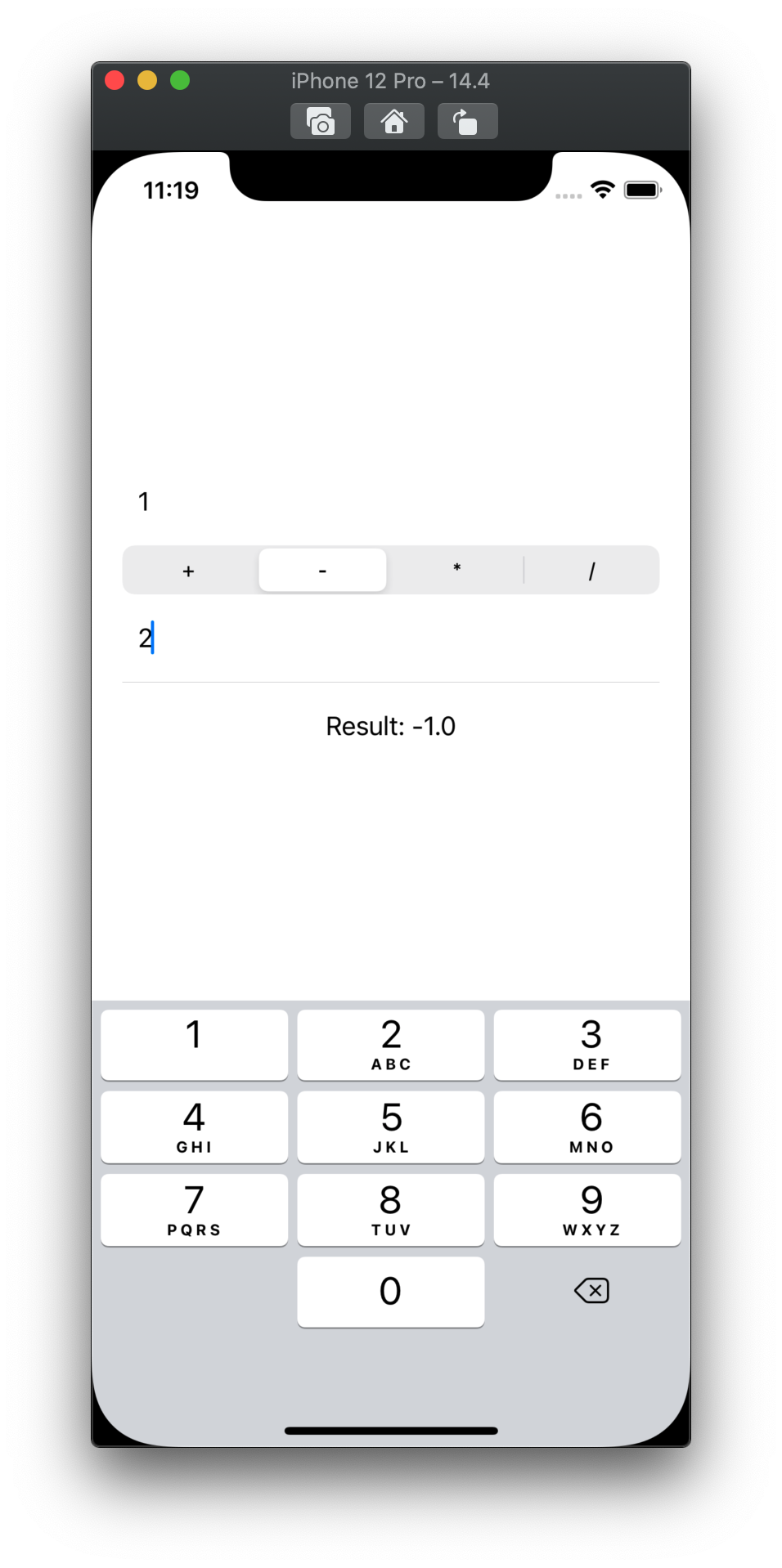### Time for some cleanup

Our shared codebase is now ready to grow, but we want to keep the maintenance of our individual apps under control.

At this point you have two quite ugly lines of code in your programs:

``````// main.swift, Line 10:
print("usage: " + name + " number1 [+ | - | / | *] number2")

// ContentView.swift, Line 24:
ForEach(["+", "-", "*", "/"], id: \.self) { op in ...
``````

Both of these lines manually list the operators we have implemented, and if we add another one to the enum Operator, they won’t be updated. Even worse we might forget to add it to one of our apps.

Let’s fix this, by adding the CaseIterable protocol to the Operator enum, which gives us `Operator.allCases`, a synthesized Array with all available operators.

``````public enum Operator: String, CaseIterable {
case plus = "+"
case minus = "-"
case divide = "/"
case multiply = "*"
}
``````

Inside the ContentView.swift change the ForEach to use the .allCases instead:

``````ForEach(Operator.allCases, id: \.self) { op in
Text(op.rawValue)
}
``````

As ForEach inside the Picker adds the operator as a tag to the Text object, we now have to change the selection property too:

``````...
@State var op: Operator = .plus
...
``````

This way can also get rid of the force-unwrap inside var result: String {..}

Inside the `main.swift` of our CLI application, you can now dynamically create the operator list in the `printUsage` function:

``````func printUsage(message: String) {
let name = URL(string: CommandLine.arguments)!
.lastPathComponent
let operators = Operator.allCases
.map(\.rawValue)
.joined(separator: " | ")
print("usage: \(name) number1 [\(operators)] number2")
print("    " + message)
}
``````

Great, do you want to add another operator? No worries, just extend the enum Operator by another case and implement it in the calculate() function 🎉

## Create more local libraries to build a dependency graph

In this step, we want to add a debug logger to our CalculatorCore. We could just use the print() method, but that wouldn’t be as much fun, right? 😄

Creating more local packages is straightforward. As before, create a folder inside Sources with the package name. In this case, it is going to be `CalculatorLogger` and it contains a single `Logger.swift` file:

``````public class Logger {

public static func warn(_ message: String) {
print("⚠️ " + message)
}

public static func debug(_ message: String) {
print("🔍 " + message)
}
}
``````

Afterwards, create a new target in the package manifest and add it as a dependency to the CalculatorCore package

``````targets: [
.target(name: "CalculatorCore", dependencies: [
"CalculatorLogger"
]),
.target(name: "CalculatorLogger"),
...
]
``````

and import it in our `calculate.swift` file:

``````import CalculatorLogger

// Calculation function using our two numbers and the operator
public func calculate(number1: Double, op: Operator, number2: Double) -> Double {
Logger.debug("Now calculating \(number1) with \(number2) using \(op)")
...
}
``````

This gives the following output when running swift run inside the CalculatorPackage directory:

``````\$ swift run Calculator 13 + 14
🔍 Now calculating 13.0 with 14.0 using plus
Result: 27.0
``````

## More to come!

This is it. If you followed along you have now created a multi-platform app using the same core logic 🚀 Some closing remarks on why this is useful:

• If we change our application, the packages won’t have to be rebuilt which gives us faster build times.
• We can work with the packages themselves, especially when adding unit tests to them, without running a full app.
• Isolation of packages takes care of keeping our code clean using visibility (e.g. public vs. internal)
• Something we haven’t explored in this post yet is parallel compilation. Imagine you are using more packages as dependencies to our CalculatorCore packages similar to the CalculatorLogger package. As these are not depending on each other, they can be built in parallel, which gives us even faster build times!

While writing this article I realized it is not possible to cover the more advanced capabilities, such as per-platform UI modules using interfaces to communicate in a VIPER pattern (which is something I am currently using in a large-scale iOS/macOS cross-platform app). Therefore I will cover advanced topics, such as how SPM can help you transitioning from UIKit/AppKit to SwiftUI using XIB files into their own packages, in a future article (make sure to follow me to get notified!).

If you would like to know more, check out my other articles, follow me on Twitter and feel free to drop me a DM. Do you have a specific topic you want me to cover? Let me know! 😃

EDIT 13.04.2021: Added an example for spin-off app for large-scale projects

UPDATE 19.04.2021: I just published the follow-up article!